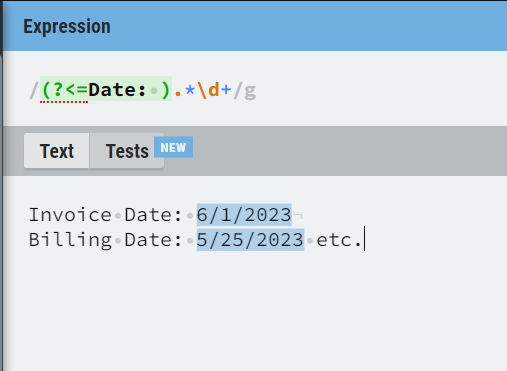# Regex match help

Hi,
I am trying to match the following reg against “Due Date:” or “Due By:”. But it is also matching
Invoice Date: 6/1/2023
Billing Date: 5/25/2023 etc.

How to fix this? Thank you for the help.

(?<=Due Date(:)?|Due By:)(\s?[A-Z]+[a-z]+\s?\d+,?\s+\d+)|(\d{1,2}/\d{1,2}/\d{4})

@A_Learner

Use the below regex expression

``````(?<=((Due Date\:?)|(Due By\:?))\s+)\d+.?\d+.?\d+
``````

Or else using the assign activity and assign the below syntax

``````System.Text.RegularExpressions.Regex.Match(yourString,"(?<=((Due Date\:?)|(Due By\:?))\s+)\d+.?\d+.?\d+").Value
``````

Hope it works!!

Try this

`(?<=Date: ).*\d+``(?<=Due \w+\: ).*`I hope it helps!!

Was able to fix based on looking at your regex. Thanks a lot.

(?<=((Due Date:?)|(Due By:?))\s+)((\d+.?\d+.?\d+)|([A-Za-z]+\s+\d{1,2},\s+\d{4}))